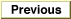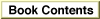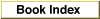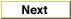# Legacy DocumentImportant: The information in this document is obsolete and should not be used for new development.Inside Macintosh: Imaging With QuickDraw /
Chapter 2 - Basic QuickDraw / Basic QuickDraw Reference
Data Structures /

### Rect

You can use a rectangle, which is a data structure of type `Rect`, to define areas on the screen and to specify the locations and sizes for various graphics operations. For example, a port rectangle represents the area of a graphics port (described on page 2-27) available for drawing.

The `Rect` data type can be defined by two points or four integers. The two points define the upper-left and lower-right corners of a rectangle; the four integers define the vertical and horizontal coordinates of the two points.

```TYPE Rect =
RECORD
CASE Integer OF            {cases: 4 boundaries or 2 corners}
0: (top:       Integer;    {upper boundary of rectangle}
left:      Integer;    {left boundary of rectangle}
bottom:    Integer;    {lower boundary of rectangle}
right:     Integer);   {right boundary of rectangle}
1: (topLeft:   Point;      {upper-left corner of rectangle}
botRight:  Point);     {lower-right corner of rectangle}
END;
```
##### Field Description
`top`
The vertical coordinate of the upper-left point of the rectangle.
`left`
The horizontal coordinate of the upper-left point of the rectangle.
`bottom`
The vertical coordinate of the lower-right point of the rectangle.
`right`
The horizontal coordinate of the lower-right point of the rectangle.
`topLeft`
The upper-left corner of the rectangle.
`botRight`
The lower-right corner of the rectangle.
Note that while the vertical coordinate appears first in this data structure, followed by the horizontal coordinate, the parameters to all QuickDraw routines expect the horizontal coordinate first and the vertical coordinate second.

See the chapter "QuickDraw Drawing" for descriptions of the QuickDraw routines you can use for calculating and manipulating rectangles for drawing purposes.